This resource function is obsolete. Use the function TorusGraph instead.

Function Repository Resource:

# TorusGraph

Create a grid graph of any dimension with the topology of a torus

Contributed by: Stephen Wolfram
 ResourceFunction["TorusGraph"][{n1,n2,…,nk}] gives the k-dimensional toroidal grid graph with n1×n2×…×nk vertices.

## Details and Options

ResourceFunction["TorusGraph"][{n1,n2,,nk},DirectedEdgesTrue] gives a directed toroidal grid graph.
TorusGraph takes the same options as Graph.

## Examples

### Basic Examples (1)

Make a 10×5 toroidal grid graph:

 In:=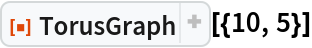Out=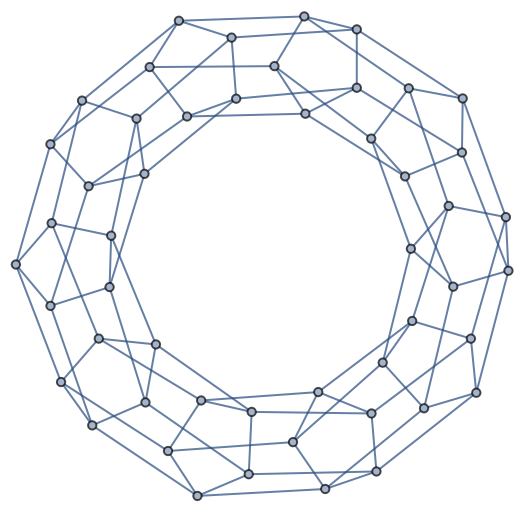### Scope (2)

Create a one-dimensional toroidal grid graph:

 In:=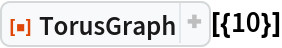Out=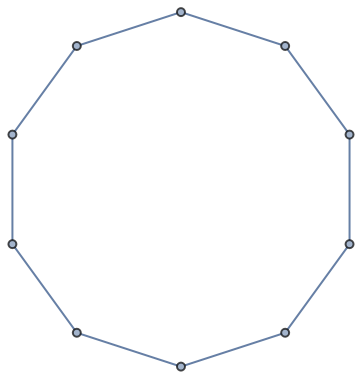Create a three-dimensional toroidal grid graph:

 In:=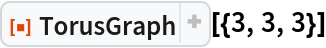Out=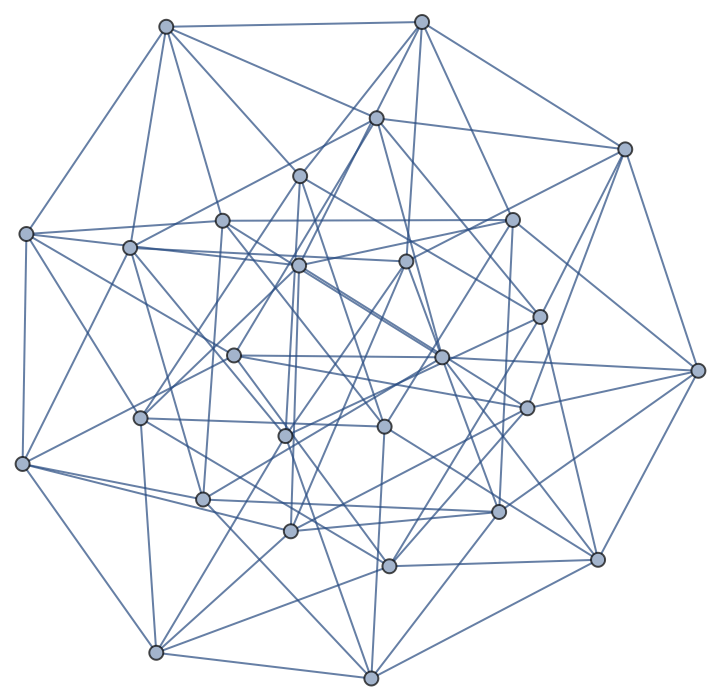Visualize the torus graph in 3D:

 In:=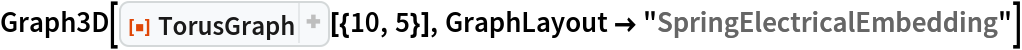Out=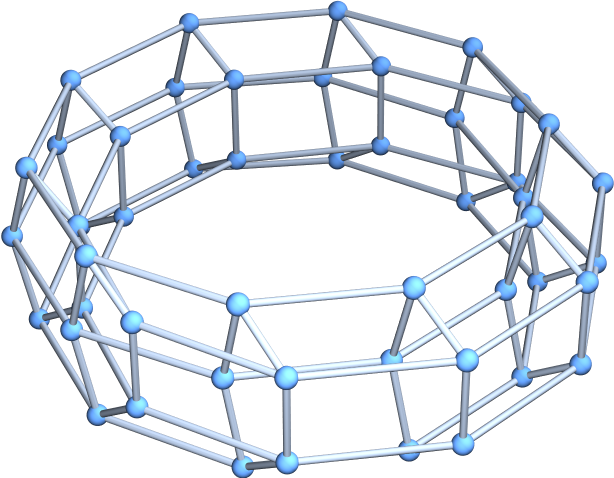### Options (1)

#### DirectedEdges (1)

Use directed edges:

 In:=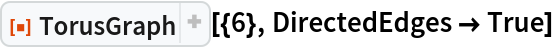Out=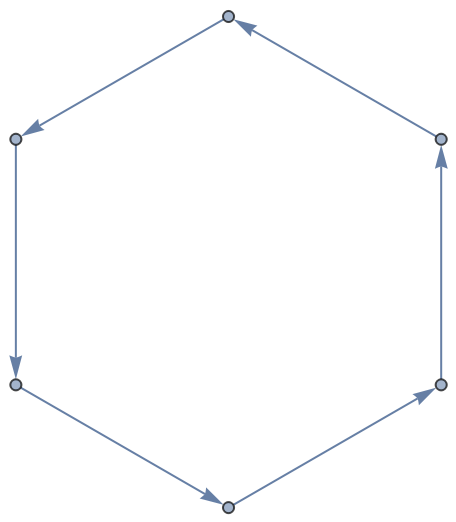In:=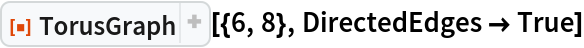Out=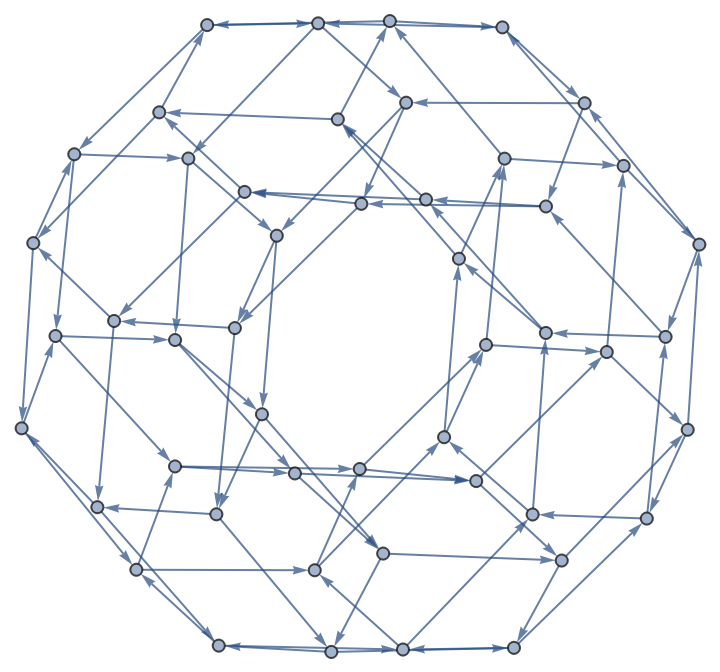### Properties and Relations (2)

Compare a grid graph to a toroidal grid graph:

 In:=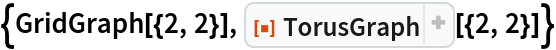Out=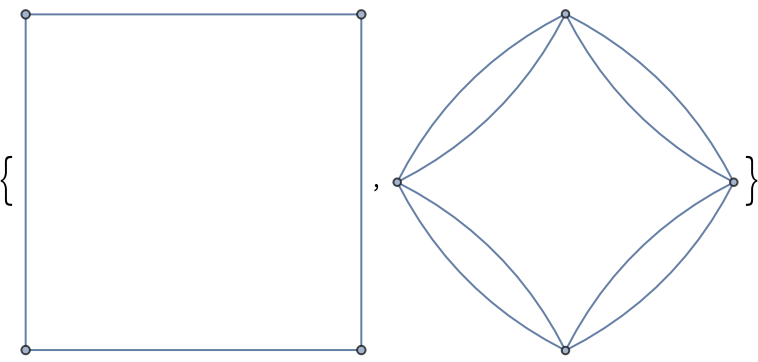In:=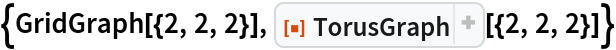Out=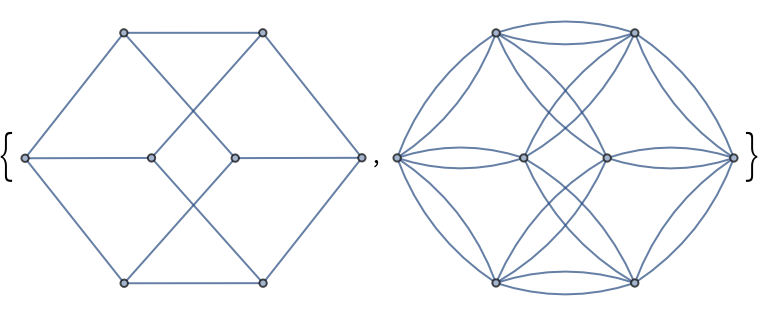A grid structure becomes increasingly visible for larger toroidal grid graphs:

 In:=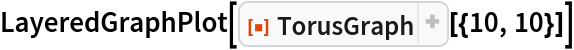Out=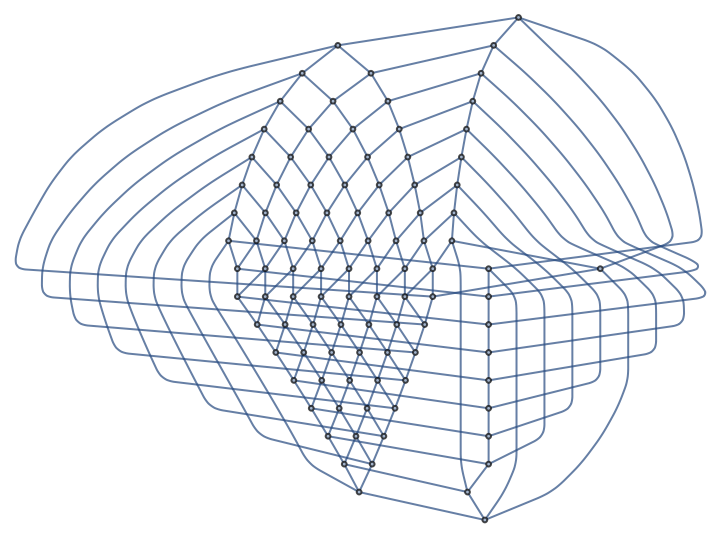## Version History

• 2.0.0 – 18 March 2020
• 1.0.0 – 04 December 2019